# HBSE 7th Class Maths Solutions Chapter 14 Symmetry InText Questions

Haryana State Board HBSE 7th Class Maths Solutions Chapter 14 Symmetry InText Questions and Answers.

## Haryana Board 7th Class Maths Solutions Chapter 14 Symmetry InText Questions

Try These (Page 272)

Question 1.
(a) Can you now tell the order fo the rotational symmetry for an equilateral triangle?
(b) How many positions are there at which the triangle looks exactly the same, when rotated about its centre by 120°?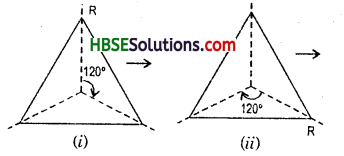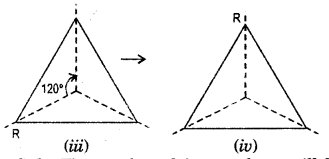Solution:
The number of times a shape will fit onto itself in one complete turn is called the order of rotation symmetry.
(a) Thus, we say that an equilateral triangle has rotational symmetry of orders.
(b) We have seen in the figure that there are three positions where it appears not to have moved.Question 2.
Which of the following shapes have rotational symmetry about the marked point ?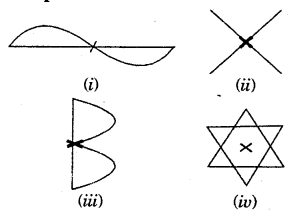Solution: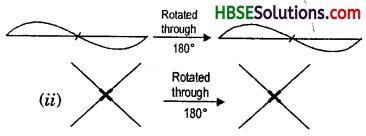Try These (Page 273)

Question 1.
Give the order of the rotational symmetry of the given figures :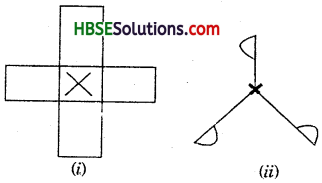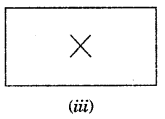Solution:
Fig. (i).
Look at the fig (i) and rotate the figure about the mark point (x). Rotate the figure (i) by 90°.
We get,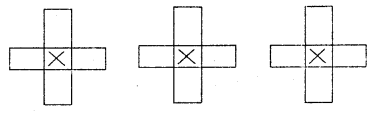And again rotate the fig. (i) by another 90°, a total of 180° we get,
Look at the fig (ii). Rotate the figure about the mark point (x). Rotate the Fig. (i) by 90°, we get
Fig. (ii) And again rotate the fig. (ii) by another 90°, a total of 180° we get,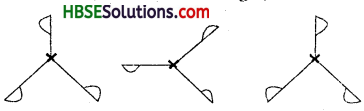Fig. (iii). Look at the fig. (iii) and, rotate the figure about the mark point (x). Rotate the figure (iii) by 90°, we get,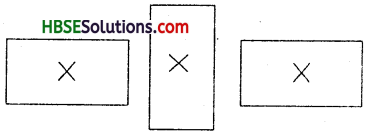And again rotate the fig. (iii) by another 90°, a total of 180°, we get,